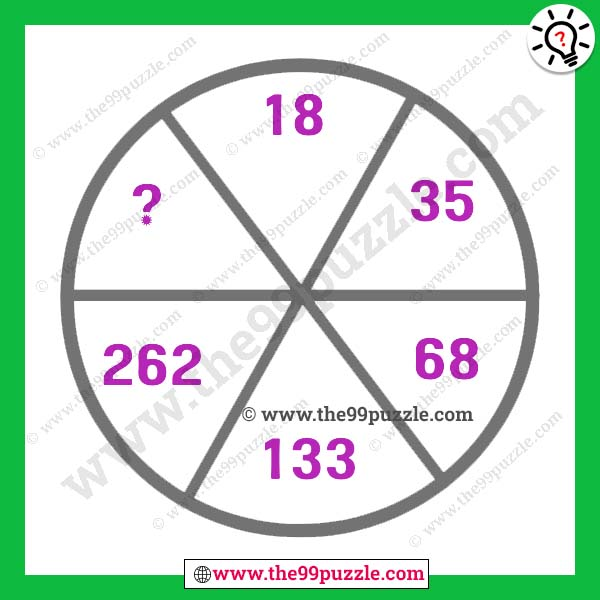# Simple mathematical tricky question with answer – Puzz286

Solving mathematical equations is very fun and interesting. Can you solve this simple mathematical tricky question? Once you know the basics of mathematics, it becomes very interesting. This type of puzzle can always challenge your brain. 90% of puzzle lovers like this logical reasoning circle math puzzle. Only a genius solves this tricky puzzle very quickly. If you want a good puzzler, you have to practice all these puzzles. So, now solve the problem and replace the question mark.###### Explanation:

18×2 = 36-1 = 35

35×2 = 70-2 = 68

68×2 = 136-3 = 133

133×2 = 266-4 = 262

262×2 = 524-5 = 519